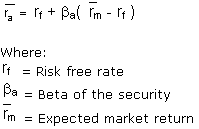# Capital Asset Pricing Model (CAPM)

Financial analysis Print Email

Meaning and definition of Capital Asset Pricing Model

The Capital Asset Pricing Model (CAPM) refers to a model that delineates the relationship between risk and expected return and what is used in the pricing of risky securities. The concept is used for pricing an individual portfolio or security. The basic idea underlying the concept is that investors are required to be compensated in two ways –

As explained by Investopedia, the CPAM states that the expected return of a portfolio or a security equals the rate on a risk free security added to a risk premium. If, in any case, this expected return does not congregate the required return, the investment should not be undertaken. The security market line plots the results of the CAPM for all individual risks.

Formula for CAPM

The general formula used for Capital Asset Pricing Model is:Example of Capital Asset Pricing Model

The concept of CAPM can be illustrated better in the following example. Let us presume that if the risk-free rate equals 3%, the beta (risk measure) of the stock is 2 and the expected market return over the period equals 10%. Then, as per the aforementioned formula, the stock is expected to produce returns equivalent to 17% (3% + 2 (10% - 3%)).

Assumptions for Capital Asset Pricing Model (CAPM)

The use of CAPM and its assumptions can be helpful in estimating the expected return of a stock. The basic assumptions of CAPM include:

1. The model aims to maximize economic utilities.

2. The results are risk-averse and rational.

3. The results are price takers. This implies that they cannot influence prices.

4. The model can lend and borrow unlimited amounts under the risk free rate of interest.

5. The model presumes that all info is available at the same time to all investors.

6. The model trades without taxation or transaction costs.

7. The model deals with securities all of which are highly divisible into small parcels.

8. The results are widely diversified across a range of investments.